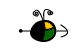Writing Equilibrium Expressions

In order to write the equilibrium expression for a system in a state of equilibrium you need to know:

• the balanced equation for the reaction
• the phases (solid, liquid, gas, or dissolved) of each species involved in the reaction.
Writing Expressions for Kc

The general equilibrium expression for a reaction:is written as: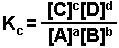The brackets "[ ]" represent the concentration of the species (moles per liter or molarity). "a, b, c, and d" represent the coefficients used to balance the equation. The "c" in Kc indicates that the value of K is determined using the concentrations of each species.

There are two cases when a species is not shown in the equilibrium expression:

• when it is a solid
• wehn it is a pure liquid or solvent
Writing Expressions for Kp

When one or more of the species in a system exists in the gaseous phase, the partial pressure of that species can be used in the equilibrium expression  Dissolved species are still expressed as moles per liter (molarity).

Top

Examples

Examples of equilibrium expressions Kc for a variety of equilibrium systems follow. When one or more gaseous substances are involved, the Kp expression is also given.

• The production of ammonia from nitrogen and hydrogen gases.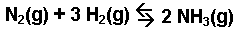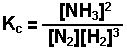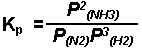• The thermal decomposition of calcium carbonate.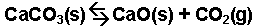• The oxidation-reduction reaction occuring between iron(III) chloride and tin(II) chloride.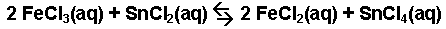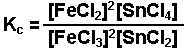• The replacement of silver ions by copper.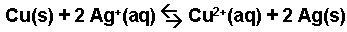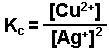• The interaction of acetic acid with water.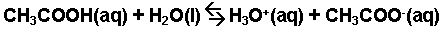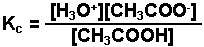• The reaction of carbon dioxide gas with a sodium hydroxide solution.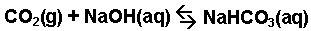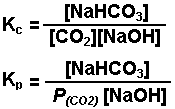• The evaporation of water.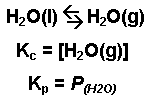Top